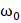# A uniform thin rod of lengthand massis hinged at a distancefrom one of the end released fron horizontal position as shown in Figure. The angular velocity of the rod as it passes the vertical position isa)b)c)d)## Question ID - 100105 :- A uniform thin rod of lengthand massis hinged at a distancefrom one of the end released fron horizontal position as shown in Figure. The angular velocity of the rod as it passes the vertical position isa)b)c)d)3537

 (b)A ring of radiusis first rotated with an angular velocityand then carefully placed on a rough horizontal surface. The coefficient of friction between the surface and the ring is. Time after which its angular speed is reduced to half is a)b)c)d)